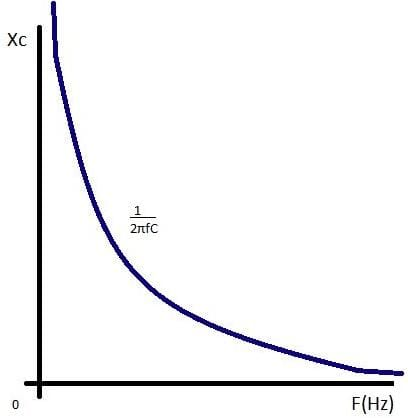NEET  >  Test: AC Applied Across Capacitor

# Test: AC Applied Across Capacitor

Test Description

## 10 Questions MCQ Test Physics Class 12 | Test: AC Applied Across Capacitor

Test: AC Applied Across Capacitor for NEET 2023 is part of Physics Class 12 preparation. The Test: AC Applied Across Capacitor questions and answers have been prepared according to the NEET exam syllabus.The Test: AC Applied Across Capacitor MCQs are made for NEET 2023 Exam. Find important definitions, questions, notes, meanings, examples, exercises, MCQs and online tests for Test: AC Applied Across Capacitor below.
Solutions of Test: AC Applied Across Capacitor questions in English are available as part of our Physics Class 12 for NEET & Test: AC Applied Across Capacitor solutions in Hindi for Physics Class 12 course. Download more important topics, notes, lectures and mock test series for NEET Exam by signing up for free. Attempt Test: AC Applied Across Capacitor | 10 questions in 10 minutes | Mock test for NEET preparation | Free important questions MCQ to study Physics Class 12 for NEET Exam | Download free PDF with solutions
 1 Crore+ students have signed up on EduRev. Have you?
Test: AC Applied Across Capacitor - Question 1

### Is it possible to use a capacitor of suitable capacitance instead of a choke coil?

Detailed Solution for Test: AC Applied Across Capacitor - Question 1

We can use a capacitor of suitable capacitance as a choke coil because the average power consumed per cycle in an ideal capacitor is zero. Therefore, like a choke coil, a condenser can reduce AC without power dissipation.

Test: AC Applied Across Capacitor - Question 2

### An a.c. voltage source E= 200 √2 sin 100t is connected across a circuit containing an a.c ammeter and a capacitor of capacitance 1μF. The reading of the ammeter is:​

Detailed Solution for Test: AC Applied Across Capacitor - Question 2

equation of alternating voltage , E = 200√2sin(100t)
compare this equation with E0sin(ωt)
so,  E0=200√2 volts
now, Erms=E0/√2
= 200√2/√2 = 200 volts .
given, capacitance of capacitor, C = 10-6 F
so, reactance of capacitor,  XC=1/ωC
=1/(10-6x100)
=104
so, the reading of Ammeter, I=Erms/XC
= 200/104 A = 20 × 10-3 A = 20mA
hence, option (b) is correct.

Test: AC Applied Across Capacitor - Question 3

### What is the unit of Capacitive Reactance Xc?​

Detailed Solution for Test: AC Applied Across Capacitor - Question 3

The opposition offered by a capacitor for the flow of A.C is called capacitive reactance.
Xc = 1/wC
it's SI unit is ohm

Test: AC Applied Across Capacitor - Question 4

The average power dissipation in pure capacitive circuit is:

Detailed Solution for Test: AC Applied Across Capacitor - Question 4

As we know,
Average power in ac circuit is given by P=Vrms​irms​cosϕ
For pure capacitive circuit ϕ=90o so P=0

Test: AC Applied Across Capacitor - Question 5

What is the natural frequency of an LC oscillator?

Test: AC Applied Across Capacitor - Question 6

What is the average power consumed/cycle in ideal capacitor.​

Detailed Solution for Test: AC Applied Across Capacitor - Question 6

Pav=(V0i0/2) cosθ
In ideal capacitor θ= -π2
Cosθ=Cosθ(-π/2)=0
Therefore
Pav=0

Test: AC Applied Across Capacitor - Question 7

Capacitive reactance of the capacitor depends upon

Detailed Solution for Test: AC Applied Across Capacitor - Question 7

Capacitive reactance (symbol XC) is a measure of a capacitor’s opposition to AC (alternating current). Like resistance it is measured in ohms, but reactance is more complex than resistance because its value depends on the frequency (f) of the signal passing through the capacitor. Reactance is also inversely proportional to the value of capacitance (C), i.e. the value of XC at any frequency will be less in larger capacitors than in smaller ones. All capacitors have infinitely high values of reactance at 0Hz, but in large capacitors, the reactance falls to a low level at much lower frequencies than in smaller capacitors. Hence, larger capacitors are preferred in low frequency applications.Test: AC Applied Across Capacitor - Question 8

he impedance of a 10 microfarad capacitor for 50 Hz ac is:

Detailed Solution for Test: AC Applied Across Capacitor - Question 8

Impedance(XC)=1/ωC
=1/ 2πf λC
=1/2πx50x10x10-6
=10-6/1000π
XC=103/π=(1000/π) Ω

Test: AC Applied Across Capacitor - Question 9

In a ac circuit with capacitance, the current

Detailed Solution for Test: AC Applied Across Capacitor - Question 9

Voltage lags current by 90° in a pure capacitive circuit. In a pure capacitive circuit, the instantaneous power may be positive or negative. As with the simple inductor circuit, the 90-degree phase shift between voltage and current results in a power wave that alternates equally between positive and negative.

Test: AC Applied Across Capacitor - Question 10

What is the capacitive reactance of 6 x 10-6 F capacitor for frequency of 106 Hz?​

## Physics Class 12

157 videos|452 docs|213 tests
 Use Code STAYHOME200 and get INR 200 additional OFF Use Coupon Code
Information about Test: AC Applied Across Capacitor Page
In this test you can find the Exam questions for Test: AC Applied Across Capacitor solved & explained in the simplest way possible. Besides giving Questions and answers for Test: AC Applied Across Capacitor, EduRev gives you an ample number of Online tests for practice

## Physics Class 12

157 videos|452 docs|213 tests

### How to Prepare for NEET

Read our guide to prepare for NEET which is created by Toppers & the best Teachers